# LASS 11 MATHS CHAPTER-7 PERMUTATION AND COMBINATION

### Exercise 7.1

Question1. How many 3-digit numbers can be formed from the digits 1, 2, 3, 4 and 5 assuming that:

(i) repetition of the digits is allowed

(ii) repetition of the digits is not allowed.

Solution :
(i) The unit place can be filled by any one of the digits 1, 2, 3, 4 and 5. Therefore the unit place can be filled in 5 ways.

Similarly, the tens place and hundreds place can be filled in 5 ways each because repetition of digits are allowed.

Total number of three digit numbers = 5 × 5 × 5 = 125

(ii) The unit place can be filled by any one of the digits 1, 2, 3, 4 and 5. Therefore the unit place can be filled in 5 ways. Hence four digits left. So the tens place can be filled in 4 ways. The hundreds place can be filled in

3 ways by the remaining three 3 digits because repetition of digits are not allowed.

∴ Total number of three digit numbers = 5 x 4 x 3= 60

Question2. How many 3-digit even numbers can be formed from the digits 1, 2, 3, 4, 5, 6 if the digits can be repeated?

Solution :
The unit place can be filled by any one of the digits 2, 4, 6. Therefore the unit place can be filled in 3 ways. Now the tens and hundreds places can be filled by any one of the digits 1, 2, 3, 4, 5, 6. Therefore, tens and hundreds places can be filled in 6 ways.

∴ Total number of three digit even numbers =3 × 6 × 6 = 108

Question3. How many four-letter codes can be formed using the first 10 letters of the English alphabet, if no letter can be repeated?

Solution :
The unit place can be filled by any one of the 10 letters of the English alphabets. So the unit place can be filled in 10 ways. The tens place can be filled in 9 ways by remaining 9 letters of the English alphabets. The hundred place can be filled in 8 ways and the thousand place can be filled in 7 ways.

∴ Total number of 4-letter code numbers =10 × 9 × 8 × 7 = 5040

Question4. How many 5-digit telephone numbers can be constructed using the digits 0 to 9 if each number starts with 67 and no digit appears more than once?

Solution :
The unit place can be filled by any one of the digits 0, 1, 2, , 4, 5, 8, 9. So the unit place can be filled in 8 ways. The tens place can be filled in 7 ways by remaining 7 digits. The hundred place can be filled in 6 ways.

∴ Total number of 5-digit telephone numbers = 8 × 7 × 6 = 336

Question5. A coin is tossed 3 times and the outcomes are recorded. How many possible outcomes are there?

Solution :
The coin is tossed first time and the number of outcomes is 2. Now the coin is tossed twice and the number of outcomes is 2 again. Also the coin is tossed thrice and the number of outcomes is 2 again.

∴ Total number of outcomes in tossing a coin 3 times = 2 × 2 × 2 = 8

Question6. Given 5 flags of different colours, how many different signals can be generated if each signal requires the use of 2 flags, one below the other?

Solution :
The upper place of the flag can be filled in 5 ways by using the 5 flags of different colours. Now the lower place of the flag can be filled in 4 ways by using the remaining 4 flags of different colours.

∴ Total number of signals = 5 × 4 = 20

### Exercise 7.2

Question1. Evaluate:

(i) 8!

(ii) (ii) 4! – 3!

Solution :
(i) 8! = 1 × 2 × 3 × 4 × 5 × 6 × 7 × 8 = 40320

(ii) 4! = 1 × 2 × 3 × 4 = 24

3! = 1 × 2 × 3 = 6

∴4! – 3! = 24 – 6 = 18

Question2.Is 3! + 4! = 7!?

Solution :
3! = 1 × 2 × 3 = 6

4! = 1 × 2 × 3 × 4 = 24

∴3! + 4! = 6 + 24 = 30

7! = 1 × 2 × 3 × 4 × 5 × 6 × 7 = 5040

∴ 3! + 4! ≠ 7!

Question3. Compute 8! / 6! × 2!

Solution : The product of the first n natural numbers is n factorial, which is written as n! and is given by, n!=n×(n−1)!

.

First consider the expression in the numerator, that is, 8!

.

According to the definition of the factorial,

8! = 8×(8−1)!

8! = 8×7!

This is further evaluated as,

8! = 8×7×6!

Now, consider the expression in the denominator, that is, 6!×2!

.

This expression can also be written in a way that is, 6!×2×1

.

Consider the expression that is to be determined and evaluated.

8! 6!×2!=8×7×6!  /  6!×2×1

8! / 6!×2! = 8×72

8! / 6!×2!=28

Therefore, the value of the expression given on evaluation is found to be 28.

Question4. If 1/6! + 1/7! = x/8!, find x

Solution :
Given:   The product of the first n natural numbers is n factorial, which is written as n! and is given by,

Question5. Evaluate n! / (n−r)!,

when

(i) n=6, r=2  (ii) n=9 ,r=5

Solution :
(i) Given: n=6, and r=2

(ii) Given: n = 9 and  r = 5

### Exercise 7.4

Question1. if nnC2,findnC2

Solution :
Given: nnC2,findnC2

Since, we know that nCa=nCb⇒a=b or m=a+b

That is,

nC2 =10C2=10! / 2!(10−2)! = 10! / 2!8!=10×9×8!  /  2×1×8!= 45

Hence, nC2=45

Question2: Determine n if

(i)2nCnC=12:1

(ii)2nCnC3 = 11:1

Solution :
(i) Given: 2nCnC=12:1

(ii) Given: 2nCnC3 = 11:1

Question3. How many chords can be drawn through 21 points on a circle?

Solution :
There are 21 points on the circumference of a circle. Since one and only one chord can be drawn by joining 2 distinct points, therefore the required number of chords given by

Question4. In how many ways can a team of 3 boys and 3 girls be selected from 5 boys and 4 girls?

Solution :
There are 5 boys and 4 girls and 3 out of 5 boys and 3 out of 4 girls have to be selected.

Number of ways of selection =

Question5. Find the number ways of selecting 9 balls from 6 red balls, 5 white balls and 5 blue balls if each selection consists of 3 balls of each colour.

Solution :Given: Total number of balls: 6 red balls, 5 white balls and 5 blue balls.

3 balls of each colour should be selected making a total of 9 balls.

Therefore,

Out of 6 red balls, 3 balls can be selected in 6C3

ways

Out of 5 white balls, 3 balls can be selected in 5C3

ways

Out of 5 blue balls, 3 balls can be selected in 5C3

ways

Therefore, by multiplication principle, number of ways of selecting 9 balls is =

= 20 × 10  × 10

= 2000

Question6. Determine the number of 5 card combinations out of a deck of 52 cards if there is exactly one ace in each combination.

Solution :
There are 4 aces and 48 other cards in a deck of 52 cards. We have to select 1 ace out of 4 aces and 4 other cards out of 48 other cards.

Question7. In how many ways can one select a cricket team of eleven from 17 players in which only 5 players can bowl if each cricket team of 11 must include exactly 4 bowlers?

Solution :There are 5 bowlers out of 17 players.

A cricket team of 11 is selected with exactly 4 bowlers.

Thus, 4 bowlers can be selected in  5C4
ways and out of remaining 12 players, 7 players are selected in 12C7

ways.

Thus, by multiplication principle, required number of ways of selecting cricket team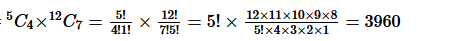Question8. A bag contains 5 black and 6 red balls. Determine the number of ways in which 2 black and 3 red balls can be selected.

Solution :
There are 5 black and 6 red balls. We have to select 2 black balls out of 5 black balls and 3 red balls out of 6 red balls.

⇒ Number of ways of selection

=  200

Question9. In how many ways can a student choose a program of 5 courses if 9 courses are available and 2 specific courses are compulsory for every student?

Solution :
There are 9 courses and number of courses to be selected are 5 in which 2 specific courses are compulsory. 3 courses out of remaining 7 courses have to be selected.

⇒ Number of ways of selection = 7 c 3

=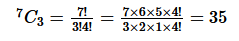### Miscellaneous Exercise

Question1. How many words, with or without meaning, each of 2 vowels and 3 consonants can be formed from the letters of the word DAUGHTER?

Solution :There are 3 vowels i.e. A, U and E and 5 consonants i.e. D, G, H, T and R in the given word.

Therefore, number of ways of selecting 2 vowels out of 3 = 3C2 =3

Number of ways of selecting 3 consonants out of 5 = 5C= 10

Thus, by multiplication principle, number of combinations = 3×10=30

.

Each of these combinations can be arranged in 5! ways.

Hence, number of different words = 30×5!=3600
.

Question2. How many words, with or without meaning can be formed using all the letters of the word EQUATION at a time so that the vowels and consonants occur together?

Solution :
There are 8 letters in the word EQUATION. In this word 5 vowels and 3 consonants. 2 vowels and 3 consonants are to be selected.

Now, 5 vowels can be arranged in 5! Ways and 3 consonants can be arranged in 3! Ways.

Also the groups of vowels and consonants can be arranged in 2! Ways.

∵ Total number of permutations = 2!×5!×3!=1440= 1440

Question3. A committee of 7 has to be formed from 9 boys and 4 girls. In how many ways can this be done when the committee consists of:

(i) exactly 3 girls

(ii) at least 3 girls

(iii) almost 3 girls?

Solution :
(i) There are 9 boys and 4 girls. 3 girls and 4 boys have to be selected.

⇒ Number of ways of selection = 4C3 × 9C4 =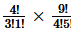=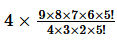= 504

(ii) We have to select at least 3 girls. So the committee consists of 3 girls and 4 boys or 4 girls and 3 boys.

⇒ Number of ways of selection =  4C× 9C4 + 4C4 × 9C3

4C3×9C4+4C4×9C3

= 504 + 84 = 588

(iii) We have to select at most 3 girls. So the committee consists of no girls and 7 boys or 1 girl and 6 boys or 2 girls and 5 boys or 3 girls and 4 boys.

∵ Number of ways of selection = 4C3  ×  9C4  +  4C2 × 9C5 + 4C1 × 9C6 + 4C0 × 9C7

= 504 + 756 + 336 + 36

= 36 + 336 + 756 + 504 = 1632

Question4. If the different permutations of all the letters of the word EXAMINATION are listed as in a dictionary, how many words are there in this list before the first word starting with E?

Solution :
In the word EXAMINATION, there are two I’s and two N’s and all other letters are different.

⇒ Number of ways of arrangement

=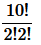= 907200

Question5. How many 6-digit numbers can be formed from the digits 0, 1 3, 5, 7 and 9 which are divisible by 10 and no digits are repeated?

Solution :
A number divisible by 10 have unit place digit 0. So digit 0 is fixed at unit place and the remaining 5 placed filled with remaining five digits in 5! ways.

∴ Required numbers = 5! = 120

Question6. The English alphabet has 5 vowels and 21 consonants. How many words with two different vowels and 2 different consonants can be formed from the alphabets?

Solution :
2 vowels out of 5 vowels and 2 consonants out of 21 consonants have to be selected and these 4 letters in 4 ways.

∵ Required number of words =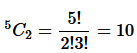Question7. In an examination, a question paper consists of 12 questions divided into two parts i.e., part I and part II containing 5 and 7 questions, respectively. A student is required to attempt 8 questions in all, selecting at least 3 from each part. In how many ways can a student select the questions?

Solution :
It is given that the question paper consists of 12 questions divided into two parts – Part I and Part II, containing 5 and 7 questions, respectively.

A student has to attempt 8 questions, selecting at least 3 from each part.

This can be done as follows.

(a) 3 questions from part I and 5 questions from part II

(b) 4 questions from part I and 4 questions from part II

(c) 5 questions from part I and 3 questions from part II

3 questions from part I and 5 questions from part II can be selected in 5C3 × 7C5  ways.

4 questions from part I and 4 questions from part II can be selected in 5C4 × 7C4 ways.

5 questions from part I and 3 questions from part II can be selected in 5C5 × 7C3 ways.

Thus, required number of ways of selecting questions

=5C3 × 7C5 + 5C× 7C5C5 × 7C3

=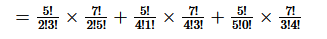= 210 + 175 + 35 = 420

Question8. Determine the number of 5-card combinations out of a deck of 52 cards is each selection of 5 cards has exactly one king.

Solution :
From a deck of 52 cards, 5-card combinations have to be made in such a way that in each selection of 5 cards, there is exactly one king.

In a deck of 52 cards, there are 4 kings.

1 king can be selected out of 4 kings in 4C1  ways.

4 cards out of the remaining 48 cards can be selected in 48C4 ways.

Thus, the required number of 5-card combinations is 4C1 × 48C4.

Question9. It is required to seat 5 men and 4 women in a row so that the women occupy the even places. How many such arrangements are possible?

Solution :
5 men and 4 women are to be seated in a row such that the women occupy the even places.

The 5 men can be seated in 5! ways. For each arrangement, the 4 women can be seated only at the cross marked places (so that women occupy the even places).

M x M x M x M x M

Therefore, the women can be seated in 4! ways.

Thus, possible number of arrangements = 4! × 5! = 24 × 120 = 2880

Question10. From a class of 25 students, 10 are to be chosen for an excursion party. There are 3 students who decide that either all of them will join or none of them will join. In how many ways can the excursion party be chosen?

Solution :

10 are chosen for an excursion party out of 25 students.

There are 2 cases since 3 students decide either all or one of them will join.

Case 1: All the 3 students join.

The remaining 7 students can be chosen out of 22 students in 22C7

ways.

Case 2: None of the 3 students join.

Thus, 10 students can be chosen in 22C10

ways.

Hence, number of ways of choosing excursion party = 22C7+22C10

= 170544 +  646646  = 817190

Question11. In how many ways can be letters of the word ASSASSINATION be arranged so that all the S’s are together?

Solution :
In the word ASSASSINATION, A appears 3 times, S appears 4 times, I appears in 2 times and N appears in 2 times. Now, 4 S’ taken together become a single letter and other remaining letters taken with this single letter.

Number of arrangements = 10! / 2!3!2!

= 151200

Get 30% off your first purchase!

X
error: Content is protected !!
Scroll to Top